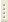06.02.2023Physical Properties of Crystals

Prof. V.N.Zverev

The course is an introduction into crystal physics and consists of two introductory parts devoted to the basics of crystallography and tensor analysis and six main chapters in which the physical phenomena in anisotropic medium are described by the example of the elastic, electrical and magnetic crystal properties. The effect of symmetry is also discussed. Elastic and electromagnetic wave propagation in crystals is detailed.

Curriculum

1. Crystal symmetry theory. Symmetry operations and elements. Point groups. System (syntony) classification. Edge and face symbols. Crystal setting. Quasicrystal concept.
2. Tensor description of crystal physical properties. Direction-dependent physical properties. Transformation of coordinates. Tensorial addition, multiplication and contraction. Characteristic surface of second rank tensor. Properties of radius vector and characteristic surface normal. Effect of symmetry on crystal properties. Neumann principle.
3. Electrical and magnetic equilibrium properties of crystals. Electrical polarization. Dielectric permeability. Crystal in uniform electrical field. Pyroelectricity and crystal symmetry. Para- and diamagnetic susceptibility. Crystal in nonuniform magnetic field. Magnetic susceptibility of powder and polycrystal.
4. Piesoelectricity.  Direct and inverse piezoelectric effect. Piezoelectric modulus tensor. Symmetry limitations. Matrix notations. Piezoelectric properties of quartz. Characteristic surface for longitudinal piezoelectric effect.
5. Elastic crystal properties. Stress and deformation tensors. Thermal expansion of crystals. Generalized Hooke law. Internal symmetry of stiffness and compliance tensors. Deformed crystal energy. Matrix notations. Neumann principle and matrix of elastic constants. Volume and linear compressibility. Elastic waves in crystals.
6. Thermodynamics of equilibrium crystal properties. Thermal, electrical and elastic properties. Thermodynamics of thermoelastic properties. Relation between coefficients measured in different conditions. Primary and secondary piezoelectric effect.
7. Properties of transfer processes. Electrical and thermal conductivity. General case of stationary heat flow. Point source heat flow. Kinetic coefficient symmetry.
8. Optical properties of crystals. Maxwell equation in anisotropic dielectric medium. Crystal birefringence. Optical indicatrix. Waves and beams, principle of duality. Fresnel ellipsoid. Effect of symmetry on crystal optical properties. Liquid crystals.